# SAT Math Multiple Choice Question 889: Answer and Explanation

### Test Information

Question: 889

4.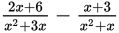Which of the following is equivalent to the rational expression given above?

• A.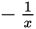• B.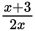• C.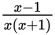• D.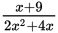Explanation:

C

Difficulty: Hard

Category: Passport to Advanced Math / Exponents

Strategic Advice: When adding fractions, you need to find a common denominator, even when the fractions contain variables. Factor the denominators to find the least common denominator (LCD).

Getting to the Answer: The original expression, after factoring each of the denominators, is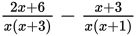. The least common denominator is x(x + 3)(x + 1). Multiply each numerator and each denominator by whatever is missing from the LCD in that particular term (the first term is missing the x + 1, and the second term is missing the x + 3). Then simplify each numerator as much as possible, and add the results by combining like terms: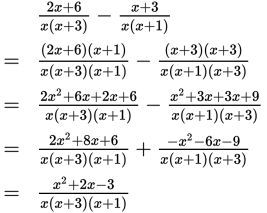This doesn't match any of the answer choices, so try to reduce further. Factor the numerator to get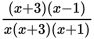. You can divide the factor (x + 3) from the numerator and denominator to get, which matches (C).